# An Intuitive Synthesis

Some art seeks to convey the beauty of a pastoral setting, the horrors of war, or the balanced sweep of a form in space. This page, which has been created to be viewed as a work of art, seeks to point to a feeling for the x and y axes of the graph below. That's the goal. That's the aesthetic destination. When you've got some vague idea of what x and y represent then you've ``got it''.

The graph space contains points that are defined in a set of visual examples (e.g. a square or a pair of lines), and a list of math-ish words, (e.g. ``a one'' or ``many''). First look at the visual examples, think of their order, then look at the table of words and think about what the words mean in relationship to the visual examples. Finally look at how these ideas fit into the graph, and therefore, what the x and y axes represent.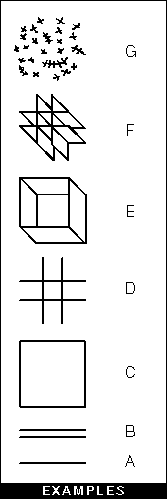The examples follow in a progression, though not one that could be stated in rational language. Try to isolate the abstract idea of this progression in your mind. See the existence of this idea beyond these examples.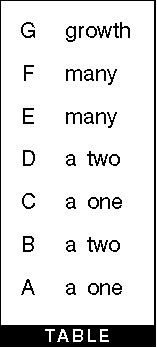The next step in understanding the concept is the table. In it the examples are related to short words or fragments such as ``a one'' or ``many''. By looking at the table and the examples you can see how A could be ``a one'' and B could could be ``a two''. What A is ``a one'' of is not stated.

C and D are ``a one'' and ``a two'' respectively and here the connection is less obvious, but because it is stated in the table, there is a connection whether apparent or not.

In the examples C looks similar to E, and D looks similar to F, yet they don't have the same words in the table. According to the table, A and C are the same. B and D are the same, and E and F are the same. The table is not describing the examples, but adding a level of meaning to them.

A through F are analogized in the table with counting statements, but G is wholly different. G is associated with ``growth'', a process.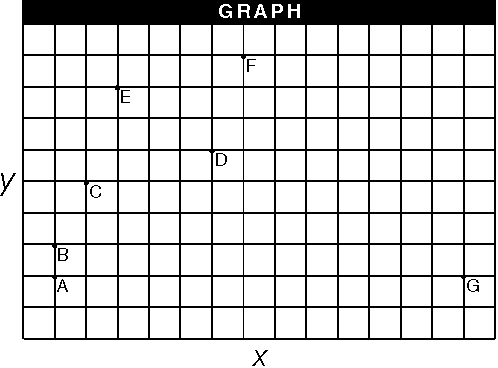The final step in understanding the intuitive synthesis is the graph. In the graph, each of the examples, now infused with additional meaning from the table, are spacially related to one another. The impression of what that spacial relationship entails is the aesthetic goal; an interplay of ideas drawn in the mind.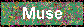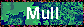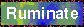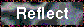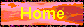